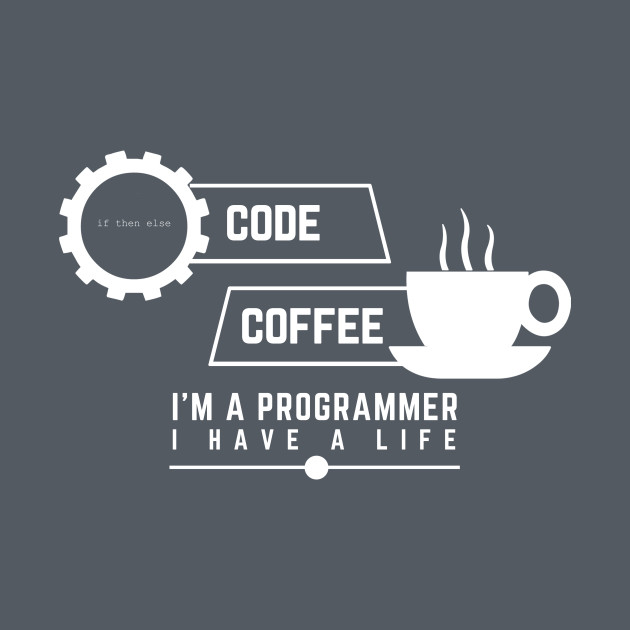# Left rotation of an array using vectors in C++

Given an array arr[] of integers and another integer D, the task is to perform D left rotations on the array and print the modified array.

Examples:

```Input: arr[] = {1, 2, 3, 4, 5, 6}, D = 2
Output: 3 4 5 6 1 2

Input: arr[] = {1, 2, 3, 4, 5, 6}, D = 12
Output: 1 2 3 4 5 6
```

## Recommended: Please try your approach on {IDE} first, before moving on to the solution.

Approach: Using vectors in C++, a rotation can be performed by removing the first element from the vector and then inserting it in the end of the same vector. Similarly, all the requried rotations can be performed and then print the contents of the modified vector to get the required rotated array.

Below is the implementation of the above approach:

 `// C++ implementation of the approach ` `#include ` `using` `namespace` `std; ` ` `  `// Function to left rotate the array by d elements ` `void` `rotate(vector<``int``> vec, ``int` `d) ` `{ ` ` `  `    ``// Push first d elements from the beginning ` `    ``// to the end and remove those elements ` `    ``// from the beginning ` `    ``for` `(``int` `i = 0; i < d; i++) { ` `        ``vec.push_back(vec); ` `        ``vec.erase(vec.begin()); ` `    ``} ` ` `  `    ``// Print the rotated array ` `    ``for` `(``int` `i = 0; i < vec.size(); i++) { ` `        ``cout << vec[i] << ``" "``; ` `    ``} ` `} ` ` `  `// Driver code ` `int` `main() ` `{ ` `    ``vector<``int``> vec = { 1, 2, 3, 4, 5, 6 }; ` `    ``int` `n = vec.size(); ` `    ``int` `d = 2; ` ` `  `    ``rotate(vec, d % n); ` ` `  `    ``return` `0; ` `} `

Output:

```3 4 5 6 1 2
```

My Personal Notes arrow_drop_upCheck out this Author's contributed articles.

If you like GeeksforGeeks and would like to contribute, you can also write an article using contribute.geeksforgeeks.org or mail your article to contribute@geeksforgeeks.org. See your article appearing on the GeeksforGeeks main page and help other Geeks.

Please Improve this article if you find anything incorrect by clicking on the "Improve Article" button below.

Article Tags :
Practice Tags :

1

Please write to us at contribute@geeksforgeeks.org to report any issue with the above content.